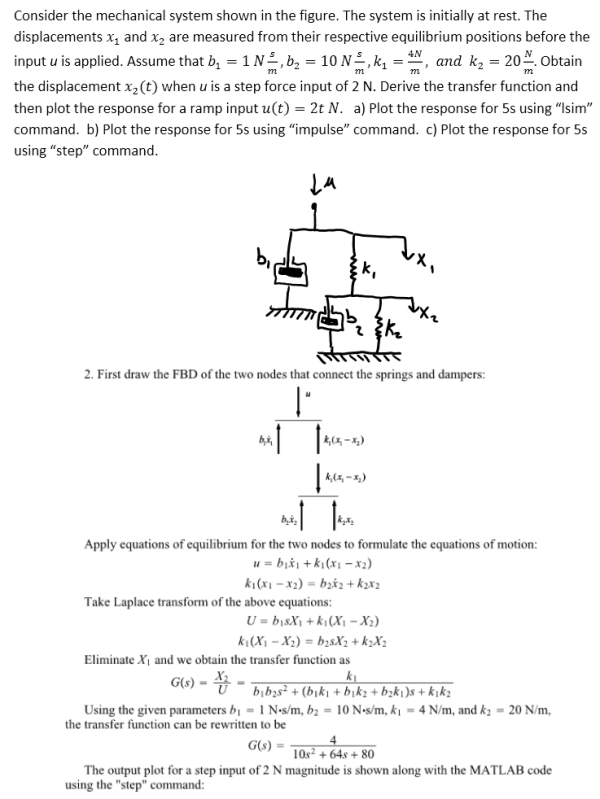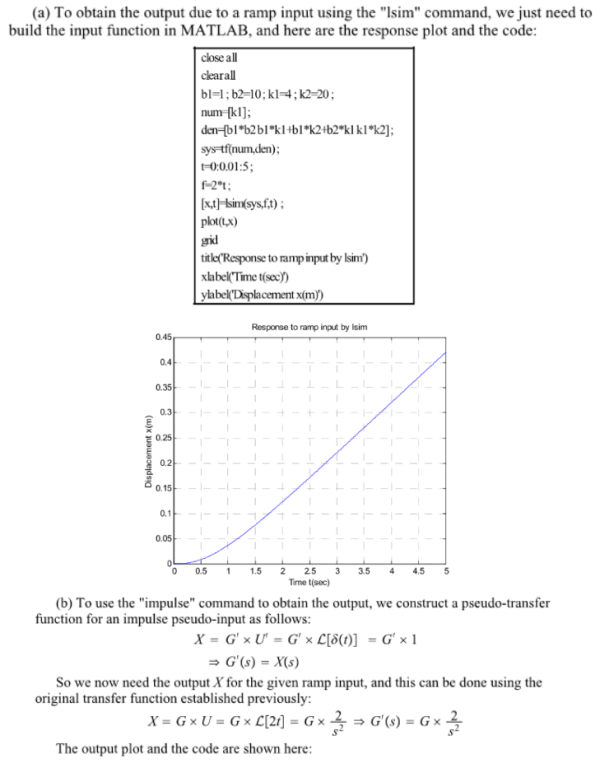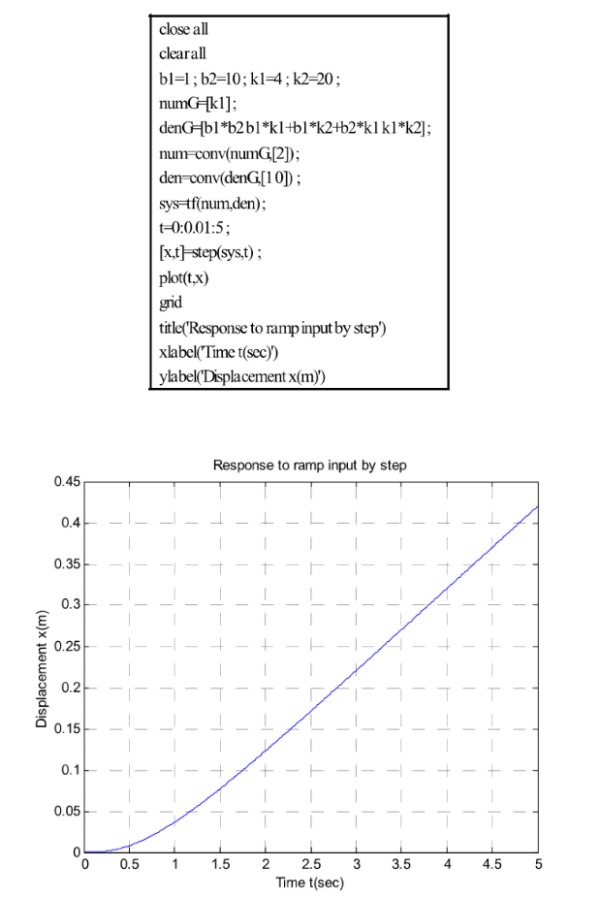Consider the mechanical system shown in the figure. The system is initially at rest. The displacements x1 and x2 are measured from their respective equilibrium positions before the input u is applied. Assume that b1=1Ns/m, b2=10Ns/m, k1=4N/m, and k2=20N/m. Obtain the displacement x2(t) when u is a step force input of 2 N. Derive the transfer function and then plot the response for a ramp input u(t)=2t N. a) Plot the response for 5s using lsim command. b) Plot the reponse for 5s using impulse command. c) Plot the response for 5s using step command.Consider the mechanical system shown in the figure. The system is initially at rest. The displacements x1 and x2 are measured from their respective equilibrium positions before the input u is applied. Assume that b1=1Ns/m, b2=10Ns/m, k1=4N/m, and k2=20N/m. Obtain the displacement x2(t) when u is a step force input of 2 N. Derive the transfer function and then plot the response for a ramp input u(t)=2t N. a) Plot the response for 5s using lsim command. b) Plot the reponse for 5s using impulse command. c) Plot the response for 5s using step command.

System Dynamics Page 2 dynamics dynamics dynamics dynamics dynamics dynamics dynamics System dynamics Page 3